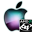ATOMS : SciColpack details

# SciColpack

Scilab interface to CSCsw/ColPack
Details
Version
0.3
Author
Stéphane Mottelet
Owner Organization
UTC
Maintainer
Stéphane Mottelet
Category
Creation Date
April 7, 2021
Source created on
Scilab 6.1.x
Binaries available on
Scilab 6.1.x:macOSLinux 64-bitWindows 64-bit
Install command
`--> atomsInstall("scicolpack")`Description
```            Scicolpack is is the Scilab interface to CSCsw/ColPack (https://github.com/CSCsw/ColPack), a
Graph Coloring Algorithm Package applied to efficient computation of sparse
Jacobian and Hessian.  When you have to compute the Jacobian of a function f or
the Hessian of f knowing its gradient g, once you know its sparsity pattern,
even if you have derived it symbolically, it may still be faster to estimate it
by using the techniques which are used by Colpack. Roughly speaking, this works
by building a graph where each vertex is associated to a column of the Jacobian
or Hessian, and an edge connects to vertices/columns if they are not
structurally orthogonal, i.e. have at least one non-zero term in a common row.
Then a proper coloring is done on this graph: at least, two adjacent vertices
cannot have the same color, but more properties of the coloring may be expected.
The coloring defines a partition of the columns under the form of p subsets and
the Jacobian (resp. Hessian) can be recovered from only p evaluation of
directional derivatives of f (resp. g). For example, for a tridiagonal matrix
the value of p is 3. In the Scilab interface these directional derivatives are
approximated by using finite differences (the toolbox allows to compute them by
using the complex step technique up to machine precision).            ```Files (5)Miscellaneous file
```
```macOS binary for Scilab 6.1.x
```
```Linux 64-bit binary for Scilab 6.1.x
```
```Windows 64-bit binary for Scilab 6.1.x
```
```Source code archive
```
```News (0)Comments (0)